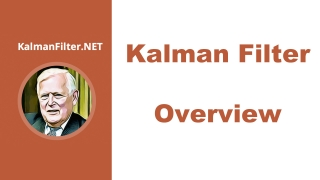Kalman Filter TutorialBeginner
Article / Blog
Theory

The Kalman Filter is an easy topic. However, many tutorials are not easy to understand. Most require extensive mathematical background which makes them difficult to understand. Also, most lack practical numerical examples.

Alex Becker decided to write a tutorial that is based on numerical examples and provides easy and intuitive explanations.

Some of the examples are from the radar world where Kalman Filtering is used extensively (mainly for the target tracking). However, the principles that are presented here can be applied to any field where estimation and prediction are required.

The tutorial includes three parts:

• Part 1 – an introduction to the Kalman Filter. This part is based on eight numerical examples. There is no requirement for a priori mathematical knowledge. All the necessary mathematical background is provided in the tutorial, and it includes terms such as mean, variance and standard deviation. That's it. You can call it "The Kalman Filter for Dummies" if you like. After reading the first part, you will be able to understand the concept of the Kalman Filter and develop “Kalman Filter intuition”. You will also be able to design a one-dimensional Kalman Filter.
• Part 2 – multidimensional Kalman Filter (Kalman Filter in matrix notation). This a bit more advanced. Most real-life Kalman Filter implementations are multidimensional and require basic knowledge of Linear Algebra (only matrix operations). The necessary mathematical background is also provided in the tutorial. The mathematical derivation of the Kalman Filter and dynamic systems modelling are also included. After reading the second part, you will be able to understand the math behind the Kalman Filter. You will also be able to design a multidimensional Kalman Filter.
• Part 3 – advanced topics. This part is for advanced readers, and it requires some mathematical background, mainly in area of statistics. Currently, this part is in the planning process. It is supposed to include Extended Kalman Filter, Unscented Kalman Filter, Kalman Filter implementation in different real-life applications and much more.

This resource is included in the following topics and journeys: